## Example:     Product of Pareto and Uniform New in mathStatica 2.0

Let random variable X ∼ Pareto(a, b) with pdf f(x):

In:=and let random variable Y ∼ Uniform(α, β) with pdf g(y):

In:=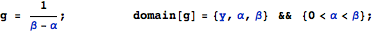Problem:  Find the pdf of V = X Y, denoted h(v).

Solution:  The solution is simply:

In3]:=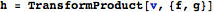Out=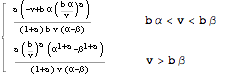A quick Monte Carlo ‘check’ of the exact solution we have just derived:
It is always a good idea to check that a theoretical solution that has been derived is consistent with pseudo-random data. To illustrate, let us suppose that (a=2, b=3, α=1, β=4). To perform a quick Monte Carlo check, we first generate 100000 pseudo-random drawings of X, and 100000 pseudo-random drawings of Y:

In:=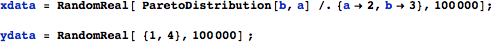We now make a frequency plot of the product of the X and Y pseudo-random data, and then compare the pseudo-random Monte Carlo solution () with the theoretical symbolic solution h(v) () derived above:

In:=Out5=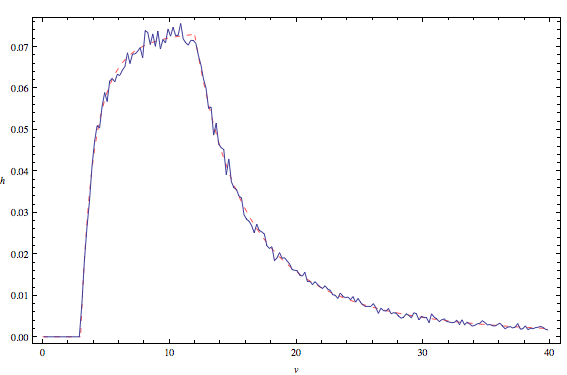Looks good!

## Example:     Product of two Standardised Normals New in mathStatica 2.0

Let random variable XN(0,1) with pdf f(x):

In:=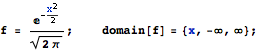The pdf of the product of two standardised Normals can then be elegantly derived via:

In:=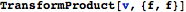Out=## Example:       Product of Two Triangulars New in mathStatica 2.0

Let random variable X ∼ Triangular(-1/2, 1, 2) with pdf f(x):

In=and let random variable Y ∼ Triangular(-1, 2, 3) with pdf g(y):

In=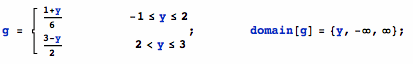The following diagram plots the pdf of both f(x) ()  and  g(y) ():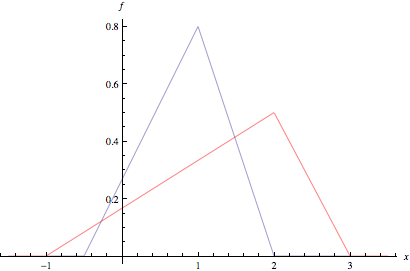Problem: Find the pdf of V = X*Y     (i.e. the pdf of the product of the two random variables).

Solution: Here is the solution pdf, say h(v):

In:=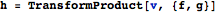Out=In:=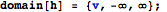The solution has a piecewise form. Here is a plot of the solution pdf:

In:=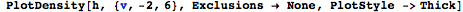Out=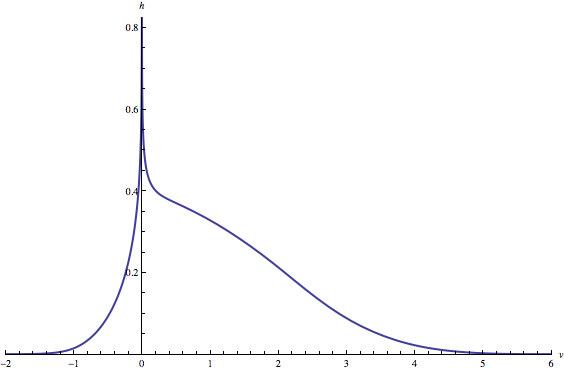A quick Monte Carlo ‘check’ of the exact solution we have just plotted:
It is always a good idea to check that a theoretical solution that has been derived is consistent with pseudo-random data. Here, we generate 100000 pseudo-random drawings of X and 100000 pseudo-random drawings of Y:

In:=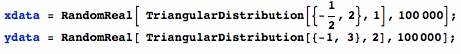We can now make a frequency plot of the product of the X and Y pseudo-random data, and then compare the pseudo-random Monte Carlo solution () with the theoretical symbolic solution h(v) () derived above:

In:=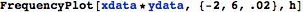Out=For the win!